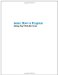# Understanding Arrays of Arrays

A multidimensional array, sometimes called a matrix, lets you process tabular data that has more than one column. For example, suppose you had the table of personal data shown in Table 6-3.

Table 6-3: A Two-Dimensional Table of Data (a Matrix)

Name

Height

SSN

Description

Neo

6'

142-1223-689

Doesn’t Neo know that life is a dream?

Morpheus

6 '3"

Morpheus? Who is Morpheus?

Trinity

5 '8"

She is a legend.

Mr. Smith

6' 2"

There are many copies of Mr. Smith.

The data in the table shown in Table 6-3 can’t easily be stored in a onedimensional array such as the sort we’ve used so far in JavaScript. (As you can see, the data in any of the columns in this table could be stored in a one-dimensional JavaScript array.)

Most languages allow you to declare multidimensional arrays. With a two-dimensional array, you would reference the data shown in Table 6-3 with two indices, the first representing the row number and the second representing the column number, as shown in Table 6-4.

Table 6-4: The Two-Dimensional Table of Data with Index Coordinates

Name

Height

SSN

Description

(0,0) Neo

(0,1) 6'

(0,2) 142-1223-689

(0,3) Doesn’t Neo know that life is a dream?

(1,0) Morpheus

(1,1) 6 '3"

(1,2)

(1,3) Morpheus? Who is Morpheus?

(2,0) Trinity

(2,1) 5 '8"

(2,2)

(2,3) She is a legend.

(3,0) Mr. Smith

(3,1) 6' 2"

(3,2)

(3,3) There are many copies of Mr. Smith.

Logically, this table should be represented by a two-dimensional array with four elements in each dimension. Then the data in each cell would be accessible using the index coordinates shown in Table 6-4. For example, if the name of the array were matrix, then the value of matrix[1,3] would be the text string Morpheus? Who is Morpheus?

 Advanced Note The expression matrix[1,3] uses square brackets, the JavaScript array operator, to reference the elements of the array. In many languages, array elements are accessed using normal parentheses, for example, matrix(1,3).

Unfortunately, we don’t have the ability to create two-dimensional arrays in JavaScript. But in many programming situations, we do have the need to easily store and retrieve tabular data that involves two (or more) dimensions (such as the data in the simple table I just showed you). What is the work around?

It’s a great thing about programming languages that if you don’t have a given facility, there’s generally a way to create it or something just about as good.

The answer is to create an array of arrays that’s the functional equivalent of a multidimensional array.

Let’s see how this works in the context of the two-dimensional array shown in Table 6-4. First, declare a one-dimensional array in the normal JavaScript fashion. For example, if the array is named matrix, you could declare a four-element single dimension array as follows:

` var matrix = new Array(4); `

Next, declare each of the four elements in the array itself as an array:

` matrix = new Array(4);  matrix = new Array(4);  matrix = new Array(4);  matrix = new Array(4); `

We now have the equivalent of a two-dimensional 4 4 array, ready to input the data from Table 6-4.

 Advanced Note In JavaScript, you don’t need to declare the dimensions of the overall array or of the array constituting an element of the overall array. In fact, the arrays making up the elements don’t need to be the same length (this is sometimes called a jagged array).

Each of the elements in the array of arrays we’ve just declared is referenced by using the square bracket array operator twice. For example, to store data in the cell 0,0, we would use a statement like this:

` matrix = "Neo"; `

 Note This notation differs slightly from what you’d expect as standard notation for a two-dimensional array, which would be matrix[0,0], but it’s just as convenient.

Listing 6-9 shows how you would declare the 4 4 two-dimensional array of arrays used to store the data shown in Table 6-4. It also shows how you can use the index values of the element arrays and the overall array to iterate through the arrays, displaying the values of each element:

` for (var i = 0; i < matrix.length; i++){     for (var j = 0; j < matrix.length; j++){          document.write ("Element (" + i + ", " + j + ") is " +             matrix[i][j] + " -- ");     }     document.write("<br>");     } `

Listing 6.9: Two-Dimensional Array Work Around` <HTML> <HEAD> <TITLE>  Two-dimensional array work around  </TITLE> <HEAD> <BODY> <SCRIPT>      var matrix = new Array(4);      matrix = new Array(4);      matrix = new Array(4);      matrix = new Array(4);      matrix = new Array(4);      matrix = "Neo";      matrix = "6";      matrix = "142 -12 2 3-689";      matrix = "Doesn't Neo know that life is a dream?";      matrix = "Morpheus";      matrix = "6:3";      matrix = "Morpheus? Who is Morpheus?";      matrix = "Trinity";      matrix = "5:8";      matrix = "She is a legend in her own Zion";      matrix = "Mr. Smith";      matrix = "6:2                                       ";      matrix = "There are many copies of Mr. Smith";      for (var i = 0; i < matrix.length; i++){      for (var j = 0; j < matrix.length; j++){          document.write ("Element (" + i + ", " + j + ") is " +             matrix[i][j] + " -- ");         }         document.write("<br>");      }     </SCRIPT> </BODY> </HTML> `If you open the page shown in Listing 6-9 in a Web browser, you’ll see the values contained in each element array displayed on its own line (see Figure 6-11).Figure 6-11: The values contained in the array of arrays are displayed.Learn How to Program Using Any Web Browser
ISBN: 1590591135
EAN: 2147483647
Year: 2006
Pages: 115
Authors: Harold Davis

Similar book on Amazon